# INA592: Max input voltage when use single supply V+=24V

Part Number: INA592
Other Parts Discussed in Thread: INA818

Hi expert,

From below figure, the max common mode input high of INA592 is 54V: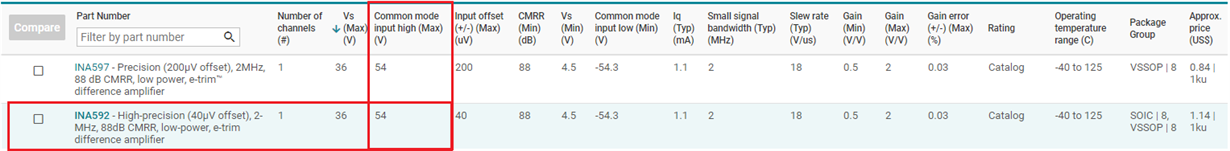However, from datasheet, the max input voltage is 3V+.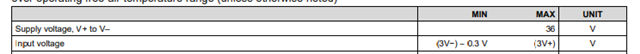So I'm a little confused, if I use single supply V+=24V, V-=GND, what' the max input voltage? Should it be 54V or 72V?

Looking forward to your reply. Thanks.

Jiandong

• Hi Jiandong,

please get the latest datasheet and read section 9.2.1.2.3.

Kai

• Hi Kai，

Thanks for tour reply. Just to confirm, in my understanding, if I use single supply V+=24V, V-=GND, the max input voltage range should be 0~72V, right?

Thanks!

• As discussed in section 9.2.1.2.3 of datasheet this depends on the gain configuration of INA592Kai

• Hi Jiandong,

As Kai pointed out, INA592 has two gain options, namely Gain=0.5 and Gain=2 (swap input and output pins in configuration).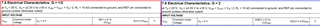With single supply V+=24V, V-=GND, and Vref=0

For Gain=1/2, assume Vref=0, then Vcm_min = 3(0-0.1V) - 2Vref = -0.3V and Vcm_max = 3(24) - 2Vref = 72V

For Gain=2, assume Vref=0, then Vcm_min = 1.5(0-0.1V) - 0.5Vref = -0.15V and Vcm_max = 1.5(24) - 0.5Vref = 36V

If you have additional questions, please let us know.

Best,

Raymond

• Hi Raymond,

Clear explanation. Many thanks!

Regards,

Jiandong.

• Hi Raymond,

My customer decide to use -5V and +24V power supply for INA592. Do you know how to calculate the supply current from -5V and 24V power rail separately? Thanks.

Regards,

Jiandong

• Hi Jiandong,

Q: Do you know how to calculate the supply current from -5V and 24V power rail separately?

I am not clear what you are asking. Are you referring to the supply requirements for -5V and 24V for operating INA592? If this is the case, I need to know your load requirements at the output of INA592.

The quiescent current is 1.5mA for both Gains of 0.5 or 2 V/V, which means that the total power consumption at non-operating state is (24-(-5)*1.5mA = 43.5mW.  If you are able to provide me with output voltage swings and driven load of INA592, maybe I am able to estimate the current requirements.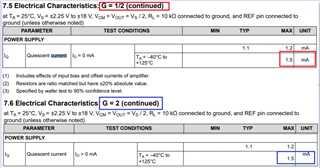Best,

Raymond

• Hi Raymond,

Thanks for your patient and clear explanation.

Customer use INA592 to measure battery voltage in battery testers. Because there are 12 series cells, each cell voltage is -0.1V to 5V during charging. Customer use 0.5V/V gain, so the output swing from -0.05 to 2.5V. Customer use INA592 to directly drive internal ADC of F280049. I didn't find the input impedance specs of ADC, so I'm not sure about the load current. Could you please help estimate the current requirements of INA592? It will helps customer to choose a suitable DC/DC power supply.

Thanks!

Regards,

Jiandong

• Hi Jiandong,

Can you email or contact me via internal email?

Here is something that I am not clear.  INA592 will be used for monitor battery charging voltage in a battery tester. Assume it is Li+ battery.

1. A typical Li+ battery has cell potential from 2.7Vdc (depleted) to 4.2Vdc (fully charge, up to 4.2Vdc). 12V of Li+ battery in series will be up to 50.4Vdc from 32.4V approximately. Li+ battery is not allowed to discharge below a low voltage threshold, say ~2.7Vdc. The above figures may vary depending on types of Li+ battery used during a test.

2. At rest state, INA592 consumes (24-(-5)*1.5mA = 43.5mW, which means that 24Vdc power supply will consume 36mW and -5Vdc consumes 7.5mW.

3.  If INA592's output is directly interface with F280049, that load current is almost negligible, which it will be less than 1mA. F280049 MCU will run a lot high current and power than INA592. Any low power 24Vdc and -5Vdc LDOs or switching power supply will meet this power requirements (including some of switched-capacitor charge pump DC/DC converter).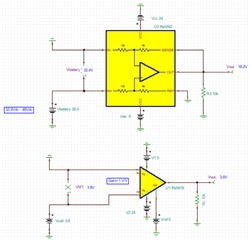Here is what I need to understand.

a. If you battery sensing voltage is measuring for 12 battery in series, then INA592's output will be at least 16.2Vdc, since Gain=0.5, see the captured image above.

b. If this is going to be measured in a single cell battery, you can use INA818, which is a low power instrumentation amplifier. Also there are other low power options the application is monitor battery voltage in a single cell configuration.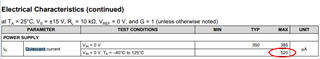Best,

Raymond